Next: Bottlenecks Up: Estimating the Speedup: Amdahl's Previous: Better Estimates for the   Contents

# Visualizing the Performance Scaling

If you're the sort of person who is thinking that all the algebraic analysis was just great to read about, but so confusing, fear not. There is indeed quite a lot to understand in all of those equations above. For example, examining our basic parallel rate equation (4.9), we see that if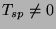it will ALWAYS prevent us from profitably reaching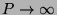for a fixed amount of work.

What, you say? You can't see that even ifgoes to zero, the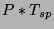diverges, and for some value of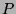(which we could easily find from calculus, if we hadn't taken a sacred oath not to put any calculus in this discussion) the overall rate begins to degrade?

Well, then. Let's Look at it. A figure is often worth a thousand equations. A figure can launch a thousand equations. A figure in time saves nine equations. Grown fat on a diet of equations, gotta watch my figure. We'll therefore examine below a whole lot of figures generated from equation (4.9) for various values of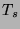,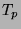, and so forth.

Let's begin to get a feel for real-world speedup by plotting (4.9) for various relative values of the times. I say relative because everything is effectively scaled to fractions when one divides by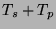as shown above. As we'll see, the critical gain parameter for parallel work is. Whenis large (compared to everything else), life could be good. Whenis not so large, in many cases we needn't bother building a beowulf at all as it just won't be worth it.

In all the figures below,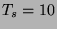(which sets our basic scale, if you like) and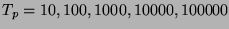. In the first three figures we just vary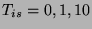for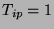(fixed). Note that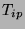is rather boring as it just adds to, but it can be important in marginal cases.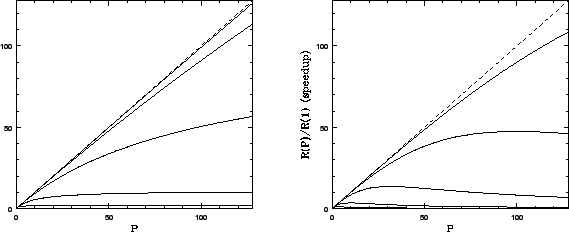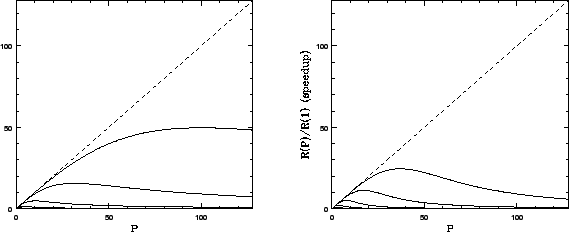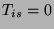(the first figure) is the kind of scaling one sees when communication times are negligible compared to computation. This set of curves (with increasingascending on the figure) is roughly what one expects from Amdahl's Law, which was derived with no consideration of IPC overhead. Note that the dashed line in all figures is perfectly linear speedup. More processors, more speed. Note also that we never get this over the entire range of, but we can often come close for small.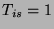is a fairly typical curve for a real'' beowulf. In it one can see the competing effects of cranking up the parallel fraction (relative to) and can also see how even a relatively small serial communications overhead causes the gain curves to peak well short of the saturation predicted by Amdahl's Law in the first figure. Adding processors past this point costs one speedup. In many cases this can occur for quite small. For many problems there is no point in trying to get hundreds of processors, as one will never be able to use them.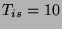(the third figure) is more of the same. Even with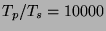, the relatively large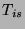causes the gain to peak well before 128 processors.

Finally, the last figure is, but this time with a quadratic dependence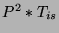. This might result if the communications required between processors is long range and constrained in some way, as our all nodes communicate every step'' example above showed. There are other ways to get nonlinear dependences of the additional serial time on, and they can have a profound effect on the per-processor scaling of the speedup.

What to get from these figures? Relatively bigis goooood.is baaaad. High powers ofmultiplying things likeor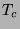are baaaaad. Real world'' speedup curves typically have a peak and there is no point in designing and purchasing a beowulf with a hundred nodes if your calculation reaches that scaling peak at ten nodes (and actually runs much, much slower on a hundred). Simple stuff, really.

With these figures under your belt, you are now ready to start estimating performance given various design decisions. To summarize what we've learned, to get benefit from any beowulf or cluster design we require a priori that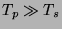. If this isn't true we are probably wasting our time. Ifis much bigger than(as often can be arranged in a real calculation) we are in luck, but not out of the woods. Next we have to considerand/orand the power law(s) satisfied by the additional-dependent serial overhead for our algorithm, as a function of problem size. If there are problem sizes where these communications times are small compared to, we can likely get good success from a beowulf or cluster design in these ranges of.

The point to emphasize is that these parameters are at least partially under your control. In many cases you can change the ratio oftoby making the problem bigger. You can changeby using a faster communications medium. You can change the power scaling ofin the communications time by changing the topology, using a switch, or sometimes just by rewriting the code to use a smarter'' algorithm. These are some the parameters we're going to juggle in beowulf design.

There are, however, additional parameters that we have to learn about. These parameters may or may not have nice, simple scaling laws associated with them. Many of them are extremely nonlinear or even discrete in their effect on your code. Truthfully, a lot of them affect even serial code in much the same way that they affect parallel code, but a parallel environment has the potential to amplify their effect and degrade performance far faster than one expects from the scaling curves above. These are the parameters associated with bottlenecks and barriers.Next: Bottlenecks Up: Estimating the Speedup: Amdahl's Previous: Better Estimates for the   Contents
Robert G. Brown 2004-05-24